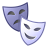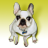# network path distance

3197
4
05-17-2010 07:26 AMNew Contributor
I need help in finding the shortest distance between two points in a shape file. That is the actual distance between the points not the straight line distance.

I have the shape files for points, routes. I need to generate a complete matrix that gives me shortest distance from one point to another this is complete problem i have. If you could help me with finding the actual distance the network distance (along the polyline) between two points I could extend it to my need.

Thank you
Tags (2)
4 RepliesNew Contributor III
If you have the Network Analyst extension, you can build your line features as a network dataset. Then, using Network Analyst, you can create an Origin-Destination (OD) analysis layer and load your points as both origins and destinations. Solving this analysis layer will provide you with a complete matrix of shortest path costs between your points, based on your underlying network dataset costs.

If you are not familiar with how to do this, then I would encourage you to read through the Network Analyst tutorial and work through its exercises. This will show you how to build network datasets and run analysis on them.New Contributor
I have did the same thing as said by you. I have around 1650 points and the OD matrix calculation is crashing for this value. Do you have any solution for this problem. any parameters so as to increase the memory. I have to find disatnce from each of this 1650 to other points.

If you have the Network Analyst extension, you can build your line features as a network dataset. Then, using Network Analyst, you can create an Origin-Destination (OD) analysis layer and load your points as both origins and destinations. Solving this analysis layer will provide you with a complete matrix of shortest path costs between your points, based on your underlying network dataset costs.

If you are not familiar with how to do this, then I would encourage you to read through the Network Analyst tutorial and work through its exercises. This will show you how to build network datasets and run analysis on them.byEsri Regular Contributor
Finding an OD for 1650 points should be very doable. When does it fail? Any error messages? How much memory do you have in the machine? How far apart are the points and do you have hierarchy in the network and using that? If it is running into memory issues, then I would suggest reading this article:
http://resources.arcgis.com/content/kbase?fa=articleShow&d=30637

Set an OriginChunkSize of 200 for your case and re-run it.

Regards,
Jay SandhuNew Contributor
I am also a newbie to all of this. I wrote up instructions for calculating the distances from one point to multiple points. I am not sure if this will be exactly what you need but it may help.

You should be able to find the instructions here: